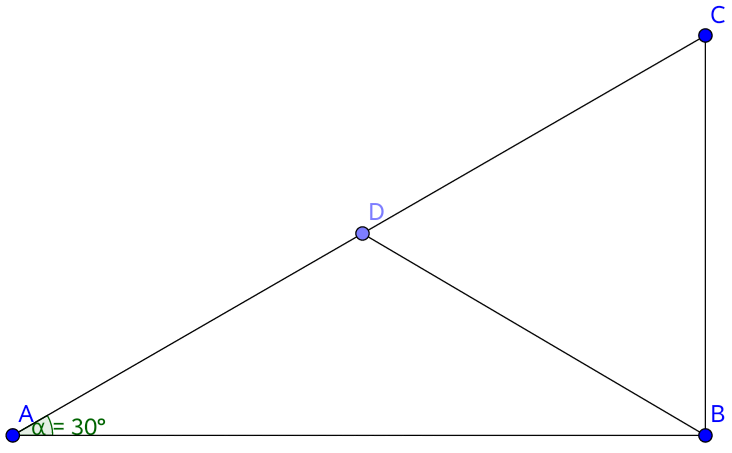# Try to solve it in a different way

Geometry Level 2In the above figure, $\triangle{ABC}$ is a right-angled triangle in which $AC$ is the hypotenuse. $\angle{BAC} = {30}^{\circ}$. $D$ is the midpoint of $AC$. Then find the value of $\angle{BDC}$.

×